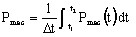# Power balance (Transient Magnetic application)

## Introduction

In Transient Magnetic application, the resolution is carried out for each time step. The power balance is carried out for a time interval defined by the user.

## Sub-systems definition: reminder

A system comprises one or more sub-systems as the one presented in the figure below.## Computation / results

For each of the sub-systems, as well as for the time interval defined by the user (Δt = t2 - t1), the following quantities are computed:

• the stored energies (instantaneous values, for t = t1 and for t = t2)
• the dissipated powers (mean values over the time interval Δt)
Attention: In the presence of symmetries and/or periodicities, the results correspond to the whole device, contrary to what is usually carried out in Flux.
Remember: The results usually correspond to the part of the device represented in the finite elements domain, with the exception of the magnetic flux in the coils, the forces/torques applied on mobile mechanical sets.

## Stored energies

For the stored energies, a summary of the computed quantities is presented in the table below.

Sub-system

Stored energy (at a moment t) (in J)

Internal

Magnetic energy stored in the computation domain: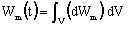, with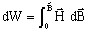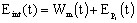Internal mechanical energy: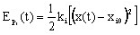Electrical

Energy stored in the coils: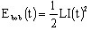Energy stored in the capacitors: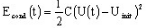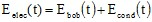Mechanical

External mechanical energy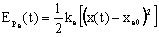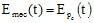Attention: In reference to the energy stored in the coils, the initial current is not taken into consideration in Flux at the present moment.

## Dissipated powers

For the dissipated powers, a summary of the calculated quantities is presented in the table below.

Sub-system

Losses (instantaneous values) (in W)

Losses (mean values) (in W)

Internal

Losses by Joule effect in solid conductors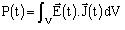Losses by Joule effect in stranded conductors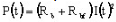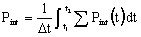Internal mechanical losses: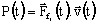(translating motion)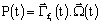(rotating motion)

Electrical Losses by Joule effect (resistive components):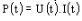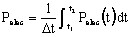Mechanical

External mechanical losses: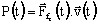(translating motion)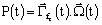(rotating motion)